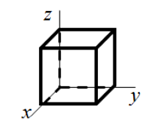# Problem: The figure below shows a cube with sides which are 3.00 m long. One corner of the cube is at the origin, and one side is along each of the Cartesian coordinate axes. What is the charge enclosed by the cube if there is a non-uniform electric field in this region of space given by E = (sin(30°)i + sin(60°)cos(30°)j + cos(60°)cos(30°)k)(5.00x N / Cm)? A. –0.597 nC B. –0.299 pC C. +0.597 nC D. +0.299 nC E. 0 C

###### FREE Expert Solution
92% (424 ratings)
###### Problem Details

The figure below shows a cube with sides which are 3.00 m long. One corner of the cube is at the origin, and one side is along each of the Cartesian coordinate axes. What is the charge enclosed by the cube if there is a non-uniform electric field in this region of space given by E = (sin(30°)i + sin(60°)cos(30°)j + cos(60°)cos(30°)k)(5.00x N / Cm)?

A. –0.597 nC

B. –0.299 pC

C. +0.597 nC

D. +0.299 nC

E. 0 C